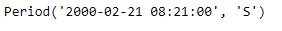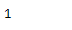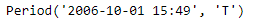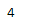# Python | Pandas Period.quarter

• Last Updated : 06 Jan, 2019

Python is a great language for doing data analysis, primarily because of the fantastic ecosystem of data-centric python packages. Pandas is one of those packages and makes importing and analyzing data much easier.

Pandas` Period.quarter` attribute return an integer value. The returned value represents the value of the quarter in the given period object.

Syntax : Period.quarter

Parameters : None

Return : integer value representing the value of quarter

Example #1: Use `Period.quarter `attribute to find the value of quarter in the given Period object.

 `# importing pandas as pd``import` `pandas as pd`` ` `# Create the Period object``prd ``=` `pd.Period(freq ``=``'S'``, year ``=` `2000``, month ``=` `2``,``                  ``day ``=` `21``, hour ``=` `8``, minute ``=` `21``)`` ` `# Print the Period object``print``(prd)`

Output :Now we will use the `Period.quarter` attribute to find out value of quarter in prd object.

 `# return value of quarter``prd.quarter`

Output :As we can see in the output, the `Period.quarter `attribute has returned 1 indicating that the given date in the prd object falls in the first quarter of the year.

Example #2: Use `Period.quarter` attribute to find the value of quarter in the given Period object.

 `# importing pandas as pd``import` `pandas as pd`` ` `# Create the Period object``prd ``=` `pd.Period(freq ``=``'T'``, year ``=` `2006``, month ``=` `10``,``                            ``hour ``=` `15``, minute ``=` `49``)`` ` `# Print the Period object``print``(prd)`

Output :Now we will use the `Period.quarter` attribute to find out value of quarter in prd object.

 `# return value of quarter``prd.quarter`

Output :As we can see in the output, the `Period.quarter `attribute has returned 4 indicating that the given date in the prd object falls in the fourth quarter of the year.

My Personal Notes arrow_drop_up